GROUP THEORY SYMBOL

# GTFatBandsPlot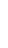GTFatBandsPlot[bands,nband,scale]
plots nband fat bands from the data in bands. scale scales the thickness of the fat bands.

## DetailsDetails

• Bands have to be calculated in a first step by means of GTBands, GTFatBandsPlot is used afterwards for a graphical representation of the fat bands. In such a plot the orbital charcter in the band at a certain k-point is expressed by the thickness of the band.
• Note, this works only for tight-binding Hamitonians with a well defined orbital characters.
• The following options can be given:
•  FrameLabel {" ", "Energy"} labels to be placed on the edges of the frame GOFermiEnergy 0 Fermi Energy GOFatBands 0 Defines the fat bands for the plot Joined False whether to join points PlotLabel "Band structure" overall label of the plot PlotRange All range of coordinates to include in plot PlotStyle Blue style of the plot
• See: W. Hergert, M. Geilhufe, Group Theory in Solid State Physics and Photonics. Problem Solving with Mathematica
• This is a new command introduced in version 1.1. A detailed description does not appear in the book.

## ExamplesExamplesopen allclose all

### Basic Examples  (1)Basic Examples  (1)

 In:=As an example the band structure of Cu is calculated.

The corresponding data is loaded from an existing dataset. Furthermore, we generate a high-symmetry path for the fcc lattice using GTBZPath and the corresponding k-mesh using GTBZLines.

 In:=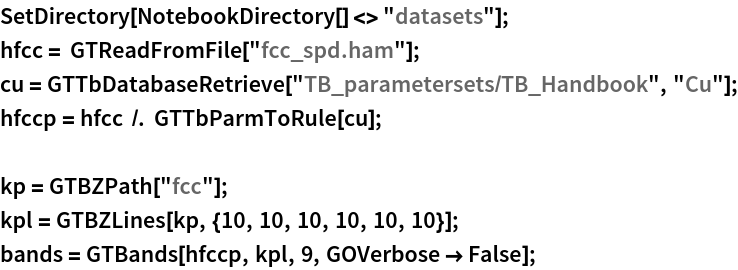The list of bands is stored in the list bands and is used to plot the band structure with GTBandsPlot.

 In:=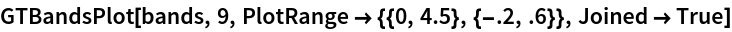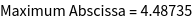Out=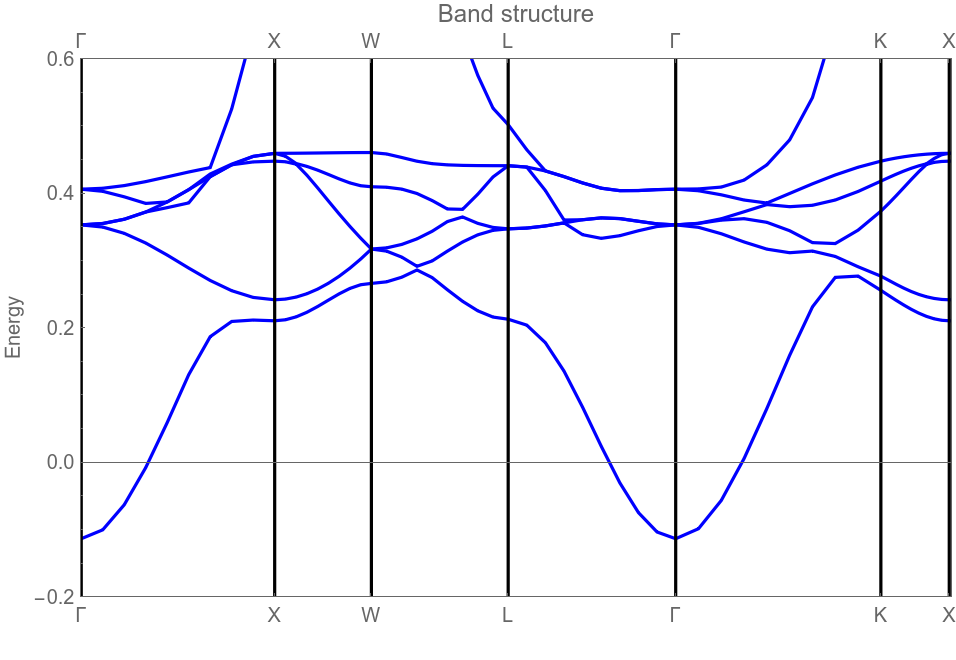To calculate fat bands the corresponding eigenvectors are needed.

 In:=To select the orbitals for the fat bands we show the form of the Hamiltonian.

 In:=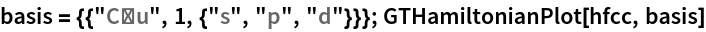Out=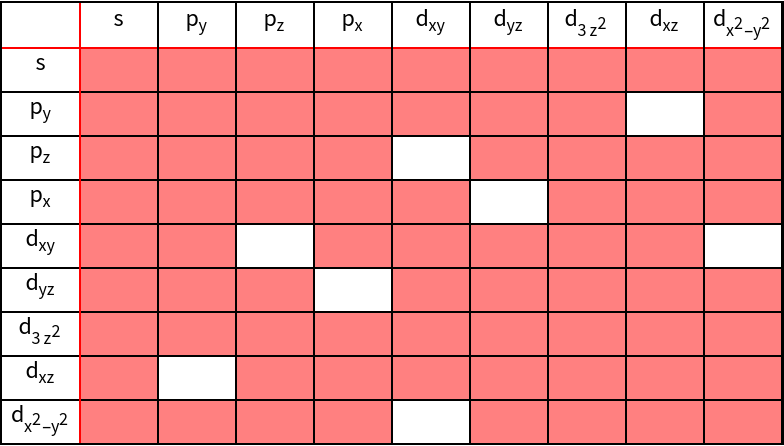We conclude that the following indices belong to the orbitals: s - 1, p -{2,3,4}, d - {5,6,7,8,9},t2g -{5,6,8}, eg -{7,9}

To highlight the d-character of the bands we use GTFatBandsPlot

 In:=Out=t2g -character

 In:=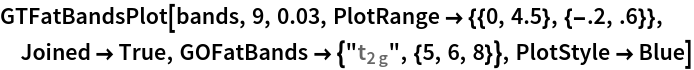Out=### Options  (3)Options  (3)

GTBandsGTBandStructureGTBZLinesGTBandsPlotGTPartialDOS